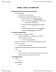# MGEA02H3 Chapter Notes - Chapter 8: Marginal Product

81 views4 pageswanyiwu and 39094 others unlocked38
Verified Note
38 documents

## Document Summary

8. 1 t he long run: no f ixed factors. Technical efficiency is when a given number of inputs are combined to maximize the level of output. cost minimization is an implication of profit maximization that firms choose the production method that produces any given level of output at the lowest possible cost. The necessary condition for cost minimization: (mpk / pk) = (mpl / pl) whenever the ratio of the marginal product of each factor to its price is not equal for all factors, there are possibilities for factor substitutions that will reduce costs. The law of diminishing returns says that, with other inputs held constant, an increase in the amount of one factor used will decrease that factor"s marginal product. profit-maximizing firms adjust the quantities of factors they use to the prices of the factors given by the market.

## Get access

\$8 USD/m\$10 USD/m
Billed \$96 USD annuallyHomework Help
Study Guides
Textbook Solutions
Class Notes
Textbook Notes
Booster Class
Class+
\$8 USD/m
Billed \$96 USD annuallyHomework Help
Study Guides
Textbook Solutions
Class Notes
Textbook Notes
Booster Class